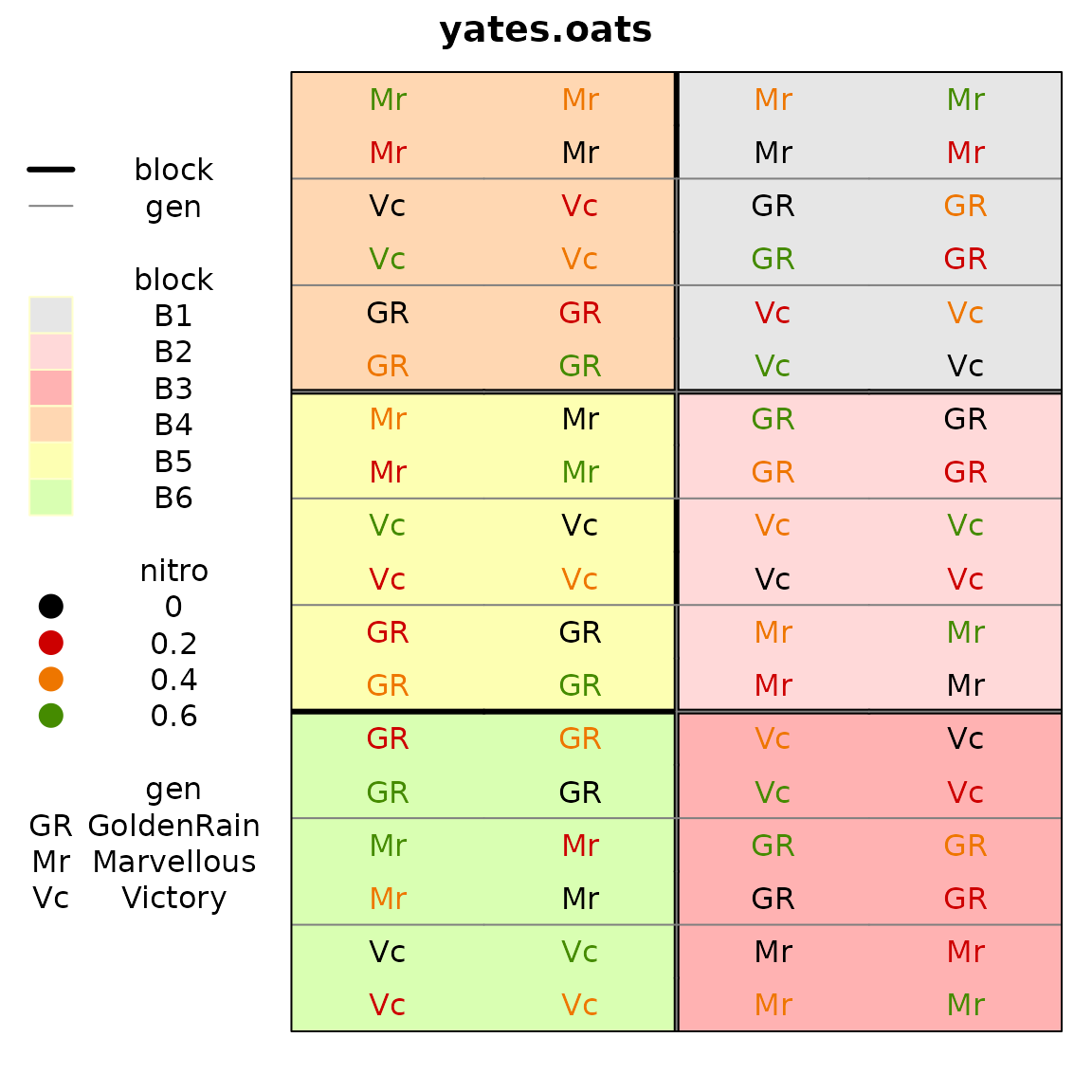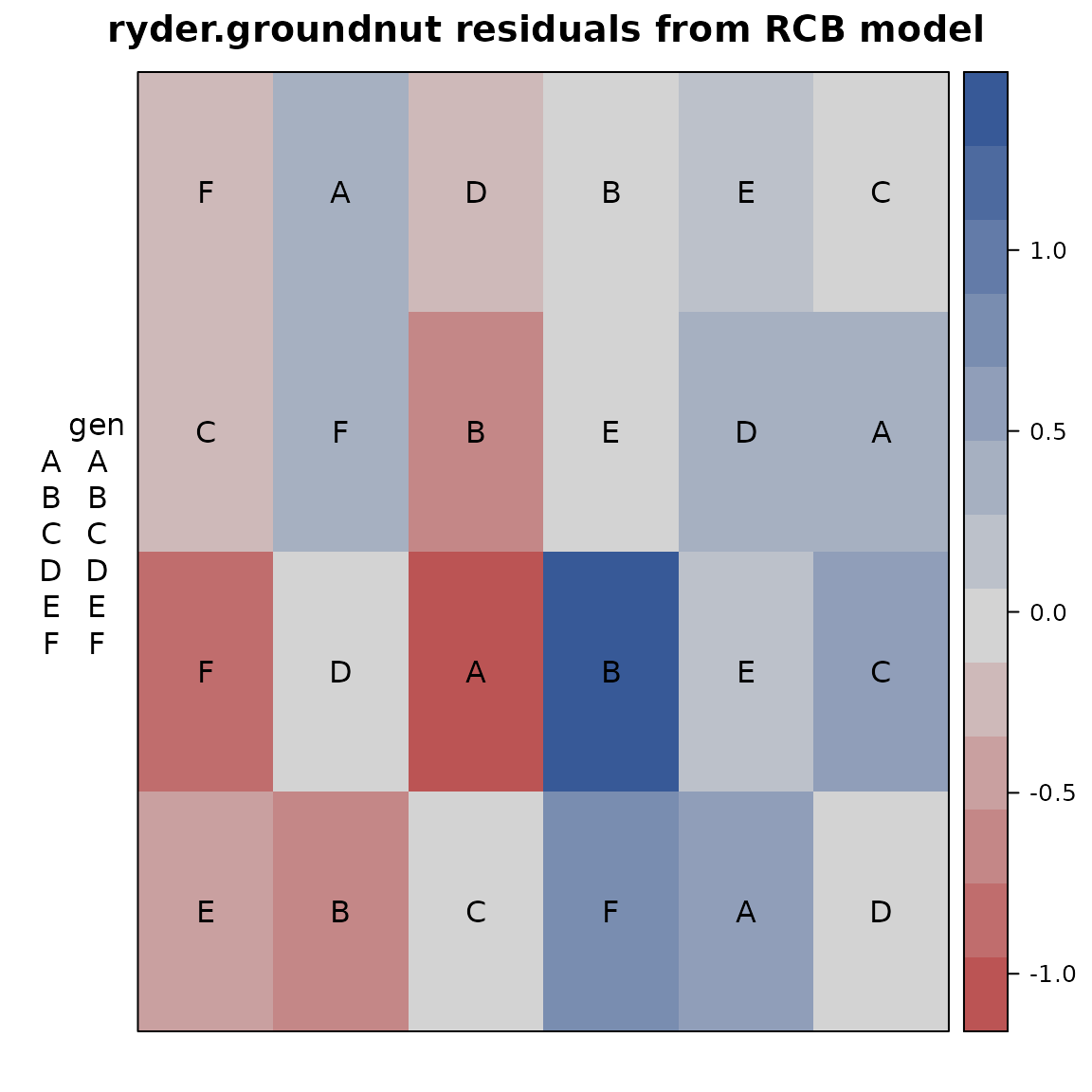# Abstract

This short note shows how to plot a field map from an agricultural experiment and why that may be useful.

# R setup

library("knitr")
knitr::opts_chunkset(fig.align="center", fig.width=6, fig.height=6) options(width=90) ## Example 1 First, a plot of the experimental design of the oats data from Yates (1935). library(agridat) library(desplot) data(yates.oats) # Older versions of agridat used x/y here instead of col/row if(is.element("x",names(yates.oats))) yates.oats <- transform(yates.oats, col=x, row=y) desplot(yates.oats, block ~ col+row, col=nitro, text=gen, cex=1, out1=block, out2=gen, out2.gpar=list(col = "gray50", lwd = 1, lty = 1))## Example 2 This next example is from Ryder (1981). Fit an ordinary RCB model with fixed effects for block and genotype. Plot a heatmap of the residuals. library(agridat) library(desplot) data(ryder.groundnut) gnut <- ryder.groundnut m1 <- lm(dry ~ block + gen, gnut) # Standard RCB model gnutres <- resid(m1)
desplot(gnut, res ~ col + row, text=gen, cex=1,
main="ryder.groundnut residuals from RCB model")Note the largest positive/negative residuals are adjacent to each other, perhaps caused by the original data values being swapped. Checking with experiment investigators (managers, data collectors, etc.) is recommended.